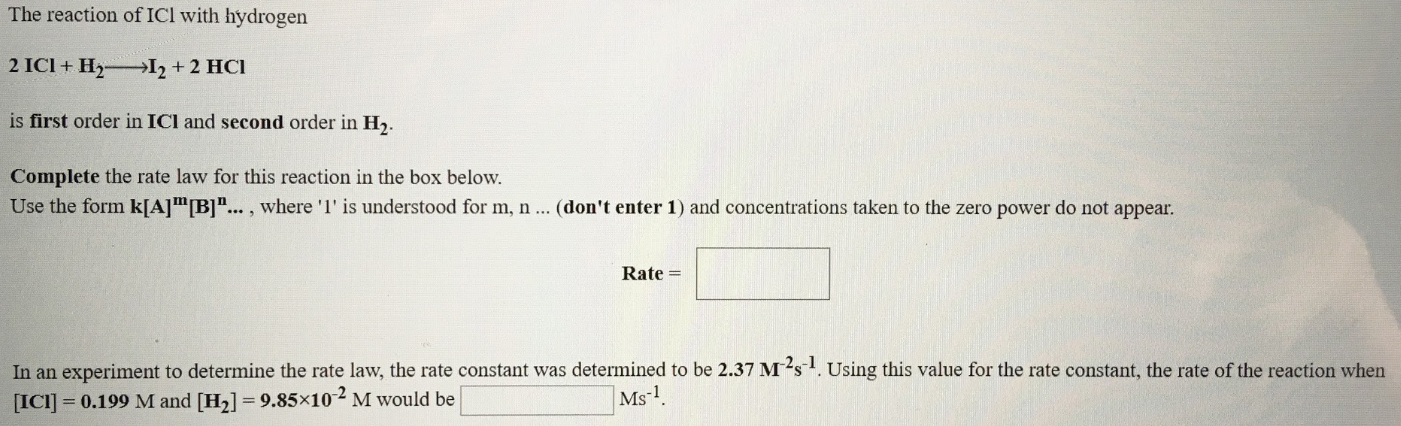# The reaction of ICl with hydrogen 2 ICl + H2 → I2 + 2 HCl is first order in ICl and second order in H2. Complete the rate law for this reaction in the box below. Use form k[A] ^m [B] ^n..., where "1" is understood for m, n ... (don't enter 1 ) and concentrations taken to the zero power do not appear. In an experiment to determine the rate law, the rate constant was determined to be 2.37 M^-2s^-1. Using this value for the rate constant, the rate of the reaction when (ICl) = 0.199 M and [H2] = 9.85x10^-2 M would be ______ Ms^-1.